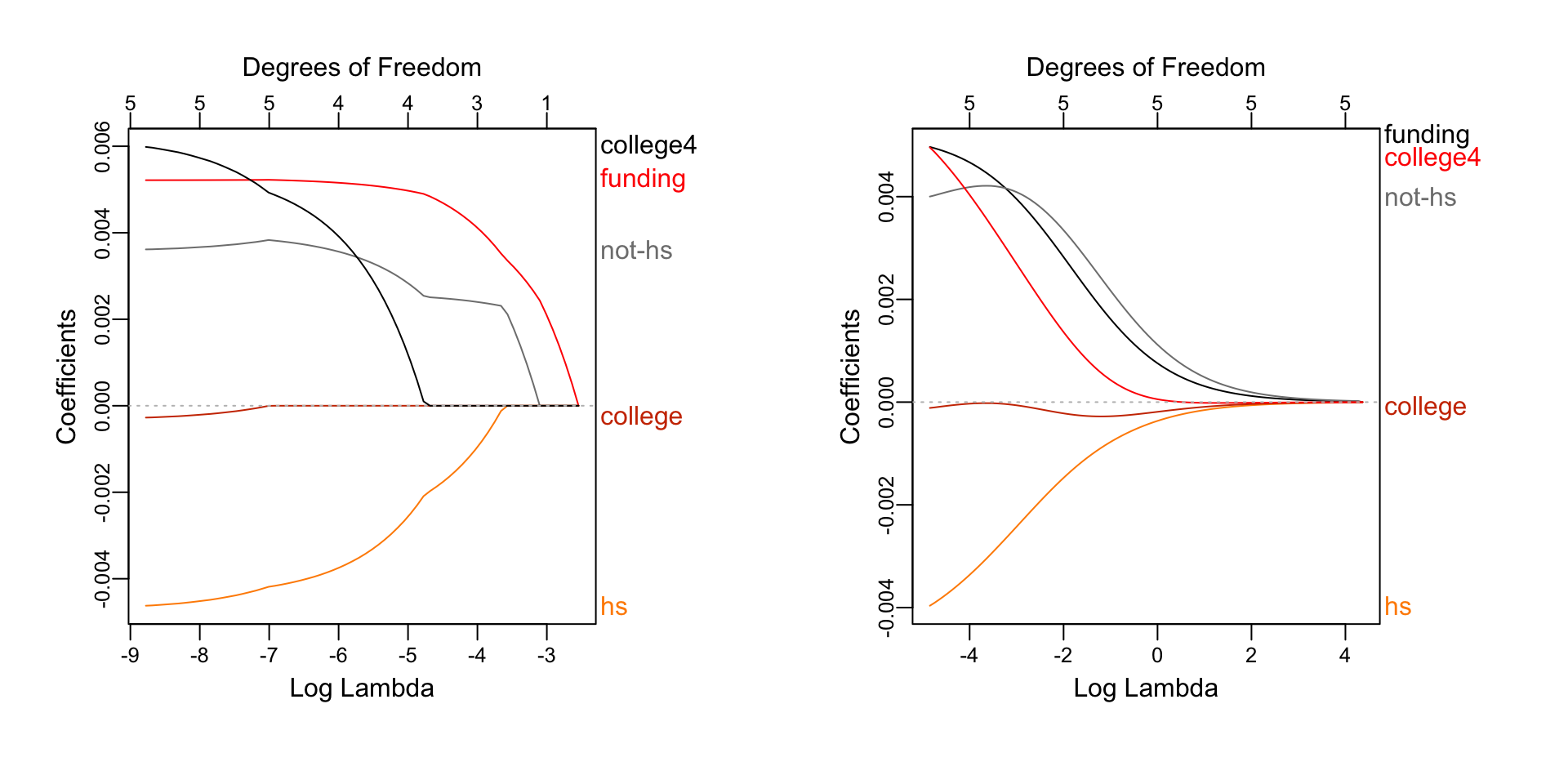We’ll reproduce the example given on page 11 of Statistical Learning with Sparsity: the Lasso and Generalizations by Hastie, Tibshirani, and Wainwright

``````data = read.csv("https://web.stanford.edu/~hastie/StatLearnSparsity_files/DATA/crime.txt",sep="\t")
names(data) = c("overall","violent","funding","hs","not-hs","college","college4")
library(glmnet)``````
``## Loading required package: Matrix``
``## Loading required package: foreach``
``## Loaded glmnet 2.0-5``
``library(plotmo) ``
``## Warning: package 'plotmo' was built under R version 3.3.2``
``## Loading required package: plotrix``
``## Warning: package 'plotrix' was built under R version 3.3.2``
``## Loading required package: TeachingDemos``
``````data\$overall = log10(data\$overall)
par(mfrow=c(1,2))
fit.lasso = glmnet(data.matrix(data[,3:7]),data\$overall)
plot_glmnet(fit.lasso,xvar="lambda")
fit.l2 = glmnet(data.matrix(data[,3:7]),data\$overall,alpha=0)
plot_glmnet(fit.l2,xvar="lambda")````````````fit.lasso = cv.glmnet(data.matrix(data[,3:7]),data\$overall)
plot(fit.lasso)
fit.l2 = cv.glmnet(data.matrix(data[,3:7]),data\$overall,alpha=0)
plot(fit.l2)``````